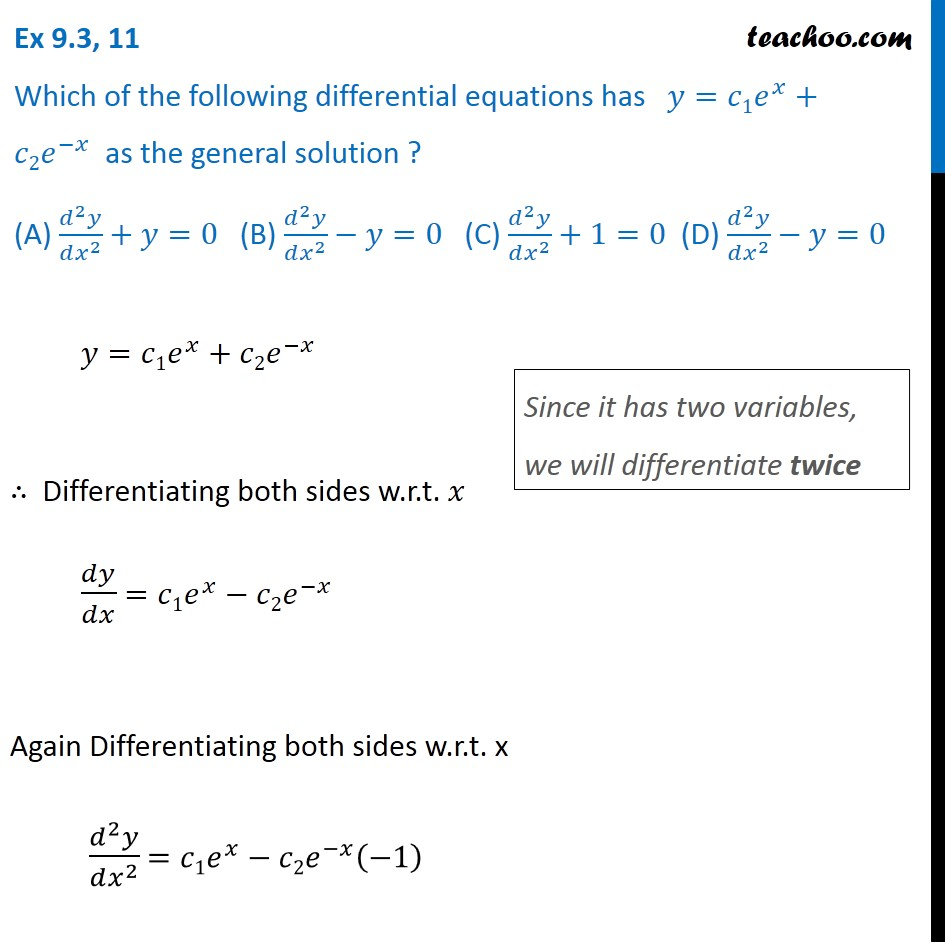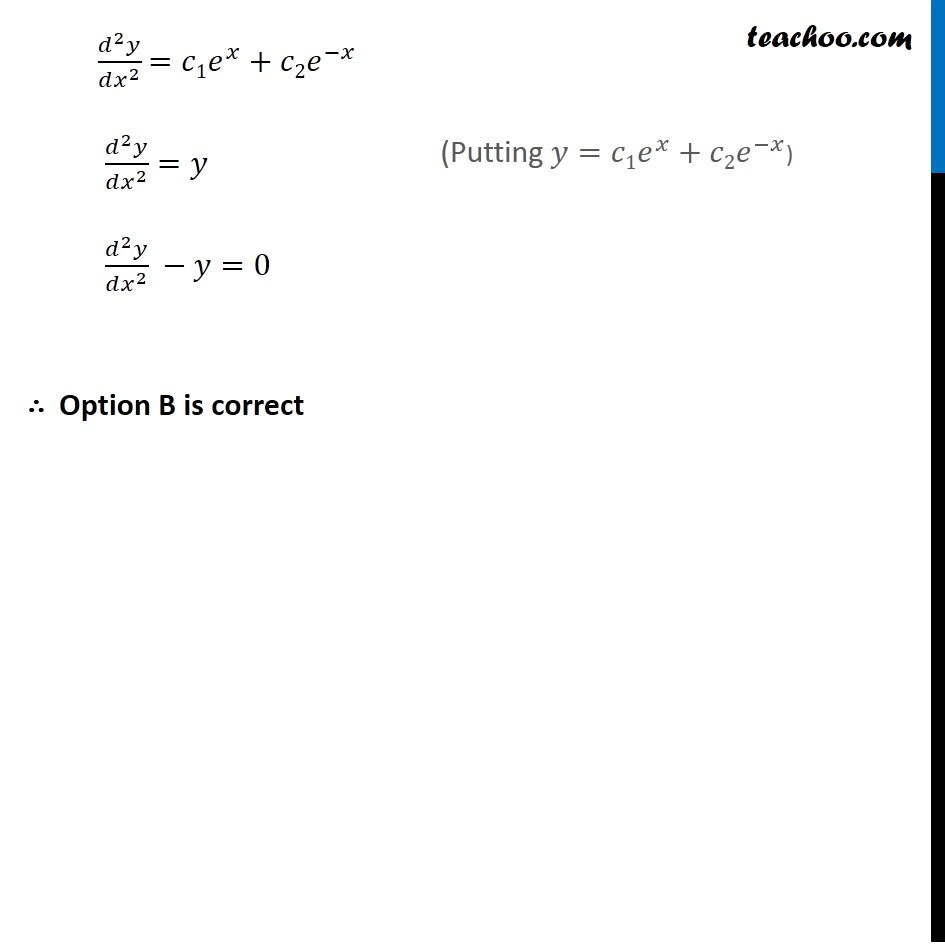Forming Differential equations

Chapter 9 Class 12 Differential Equations
Serial order wiseLearn in your speed, with individual attention - Teachoo Maths 1-on-1 Class

### Transcript

Question 11 Which of the following differential equations has 𝑦=𝑐1𝑒^𝑥+𝑐2𝑒^(−𝑥) as the general solution ? (A) (𝑑^2 𝑦)/(𝑑𝑥^2 )+𝑦=0 (B) (𝑑^2 𝑦)/(𝑑𝑥^2 )−𝑦=0 (C) (𝑑^2 𝑦)/(𝑑𝑥^2 )+1=0 (D) (𝑑^2 𝑦)/(𝑑𝑥^2 )−𝑦=0 𝑦=𝑐1𝑒^𝑥+𝑐2𝑒^(−𝑥) ∴ Differentiating both sides w.r.t. 𝑥 𝑑𝑦/𝑑𝑥 =𝑐1𝑒^𝑥−𝑐2𝑒^(−𝑥) Again Differentiating both sides w.r.t. x (𝑑^2 𝑦)/(𝑑𝑥^2 ) =𝑐1𝑒^𝑥−𝑐2𝑒^(−𝑥) (−1) Since it has two variables, we will differentiate twice (𝑑^2 𝑦)/(𝑑𝑥^2 ) =𝑐1𝑒^𝑥+𝑐2𝑒^(−𝑥) (𝑑^2 𝑦)/(𝑑𝑥^2 )=𝑦 (𝑑^2 𝑦)/(𝑑𝑥^2 ) −𝑦=0 ∴ Option B is correct (Putting 𝑦=𝑐1𝑒^𝑥+𝑐2𝑒^(−𝑥))